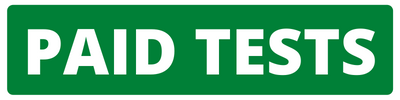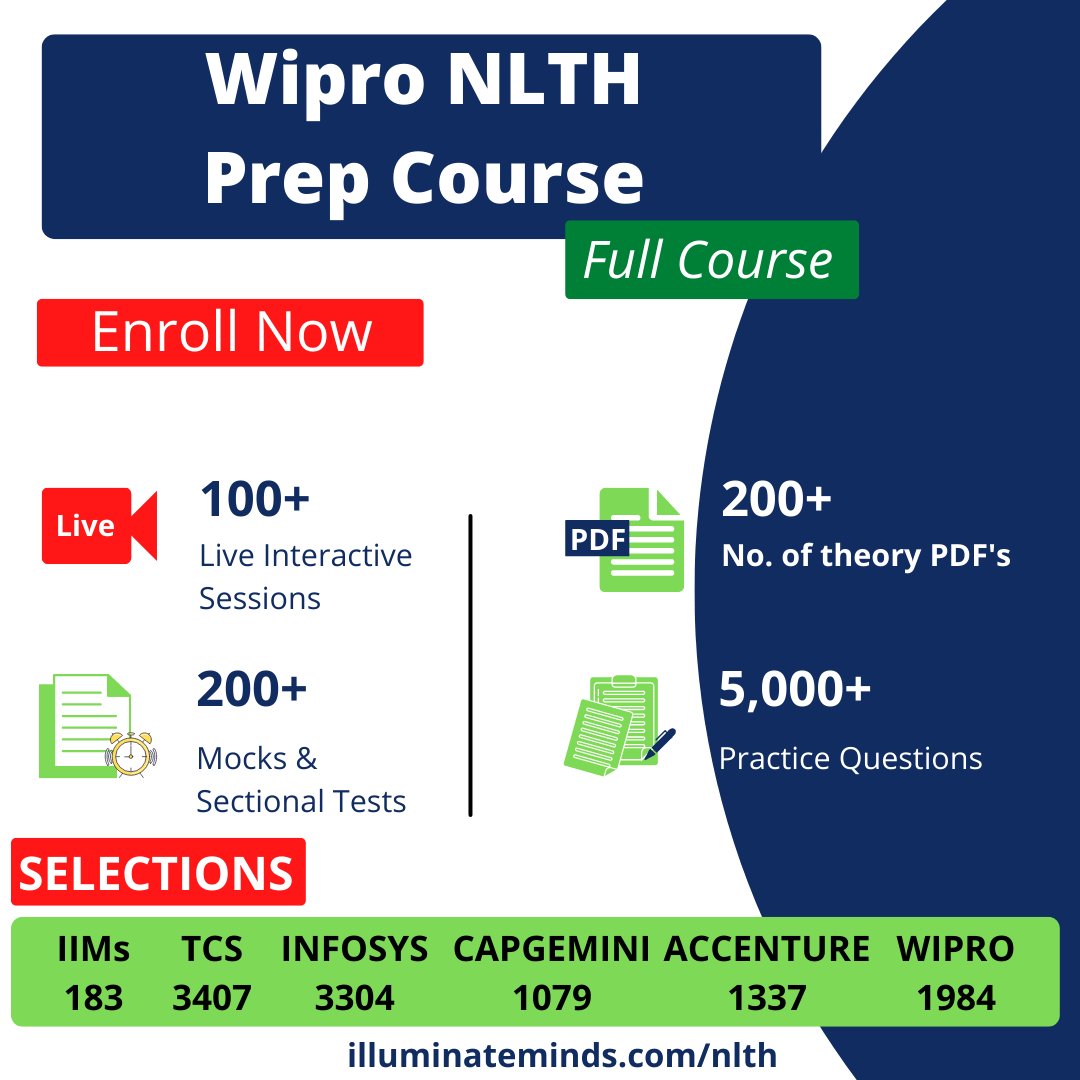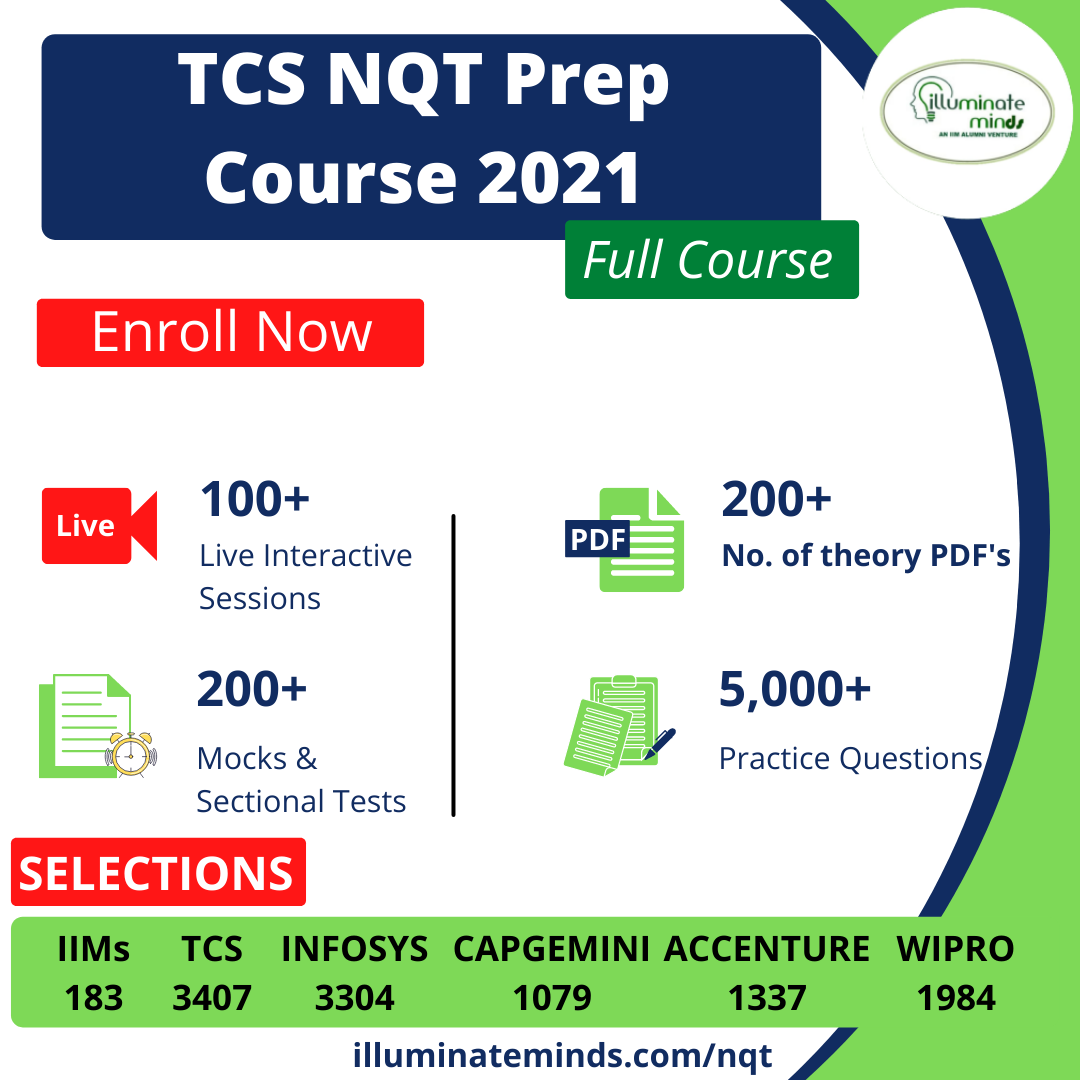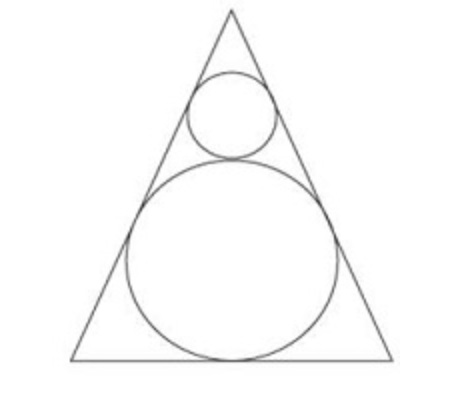CAT | Geometry

## CAT | GeometryJobs By Batch
Jobs By Location
Jobs By Degree
Jobs By Branch
IT Jobs
Internships
Govt. Jobs• ##### Zoho off campus drive 2022/ 2023 | Multiple Hirings | Any Graduates/ Post Graduates, BE, B.Tech, MBA, MCA, ME, M.Tech | 2019, 2020, 2021, 2022, 2023 Batch | Multiple Location• ##### Original Questions of IT Companies| All Topics### Quiz Begins Here

Q #1
:

#### CAT Geometry: Obtuse Triangles - Integers

x, y, z are integer that are side of an obtuse-angled triangle. If xy = 4, find z.

+

Q #2
:

#### CAT Geometry: Isosceles Triangles - Integers

How many isosceles triangles with integer sides are possible such that sum of two of the side is 12?

+

Q #3
:

#### CAT Geometry: Triangles - Area

Sides of a triangle are 6, 10 and x for what value of x is the area of the ? the maximum?

+

Q #4
:

#### CAT Geometry: Equilateral triangle and circle

Two circles are placed in an equilateral triangle as shown in the figure. What is the ratio of the area of the smaller circle to that of the equilateral triangle+

Q #5
:

#### CAT Geometry: Triangles - Perimeter

Perimeter of a ? with integer sides is equal to 15. How many such triangles are possible?

+